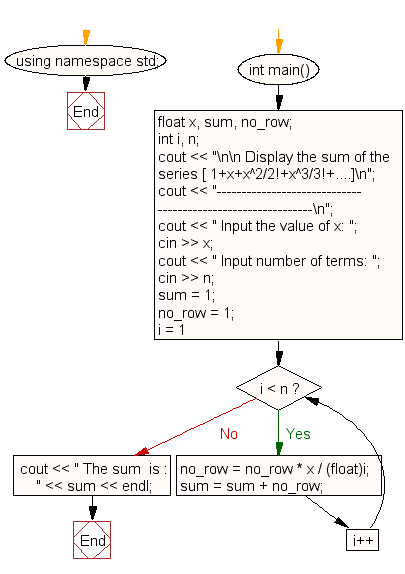﻿ C++ : Display the sum of the series 1+x+x^2/2!+x^3/3!+....

# C++ Exercises: Display the sum of the series 1+x+x^2/2!+x^3/3!+....

## C++ For Loop: Exercise-24 with Solution

Write a program in C++ to display the sum of the series [ 1+x+x^2/2!+x^3/3!+....].

Sample Solution:

C++ Code :

``````#include <iostream>
using namespace std;

int main()
{
float x, sum, no_row;
int i, n;
cout << "\n\n Display the sum of the series [ 1+x+x^2/2!+x^3/3!+....]\n";
cout << "------------------------------------------------------------\n";
cout << " Input the value of x: ";
cin >> x;
cout << " Input number of terms: ";
cin >> n;
sum = 1;
no_row = 1;
for (i = 1; i < n; i++)
{
no_row = no_row * x / (float)i;
sum = sum + no_row;
}
cout << " The sum  is : " << sum << endl;
}
``````

Sample Output:

``` Display the sum of the series [ 1+x+x^2/2!+x^3/3!+....]
------------------------------------------------------------
Input the value of x: 3
Input number of terms: 5
The sum  is : 16.375
```

Flowchart:C++ Code Editor: